Courses

Fill in the Blanks: Heat & Thermodynamics | JEE Advanced Notes | Study Physics 35 Years JEE Main & Advanced Past year Papers - JEE

JEE: Fill in the Blanks: Heat & Thermodynamics | JEE Advanced Notes | Study Physics 35 Years JEE Main & Advanced Past year Papers - JEE

The document Fill in the Blanks: Heat & Thermodynamics | JEE Advanced Notes | Study Physics 35 Years JEE Main & Advanced Past year Papers - JEE is a part of the JEE Course Physics 35 Years JEE Main & Advanced Past year Papers.
All you need of JEE at this link: JEE

Q.1. One mole of a mono-atomic ideal gas is mixed with one mole of a diatomic ideal gas. The molar specific heat of the mixture at constant volume is .......

Ans. 4 cal.

Solution.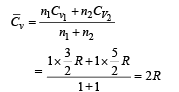Q.2. The variation of temperature of a material as heat is given to it at a constant rate is shown in the figure. The material is in solid state at the point O. The state of the material at the point P is ........

Ans. Partly solid and partly liquid

Solution.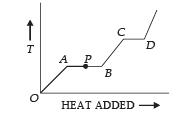Q.3. During an experiment, an ideal gas is found to obey an additional law VP2 =constant, The gas is initially at a temperature T, and volume V. When it expands to a volume 2V, the temperature becomes..........

Ans. √2T

Solution. PV = RT (Ideal gas equation)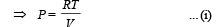Given that VP2 = const ... (ii)

From (i) and (ii)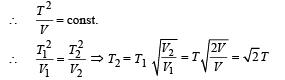Q.4. 300 grams of water at 25° C is added to 100 grams of ice at 0°C. The final temperature of the mixture is .....°C.

Ans. 0°C

Solution. The heat required for 100 g of ice at 0° C to change into water at 0°C = mL = 100 × 80 ×4.2 = 33,600 J          ... (i)

The heat released by 300g of water at 25°C to change its temperature to 0°C = mcΔT = 300 × 4.2 × 25 = 31,500 J  ... (ii)

Since the energy in eq. (ii) is less than of eq. (i) therefore the final temperature will be 0°C.

Q.5. The earth receives at its surface radiation from the sun at the rate of 1400 W m–2. The distance of the centre of the sun from the surface of the earth is 1.5 × 1011 m and the radius of the sun is 7 × 108 m. Treating the sun as a black body, it follows from the above data that its surface temperature is...........K.

Ans. 5803K

Solution. The energy received per second per unit area from Sun at a distance of 1.5 × 1011 m is 1400 J/sm2. The total energy released by Sun/per second.

= 1400 × 4π × (1.5 × 1011)2.

∴ The total energy released per second per unit surface area of the Sun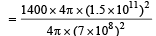This energy E is also equal to E = σT4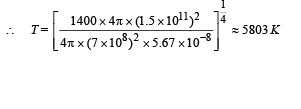Q.6. A solid copper sphere (density r and specific heat c) of radius r at an initial temperature 200 K is suspended inside a chamber whose walls are at almost 0K. The time required for the temperature of the sphere to drop to 100K is ....................

Ans. 1.71ρrc

Solution.The energy emitted per second when the temperature of the copper sphere is T and the surrounding temperature T0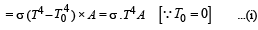We know that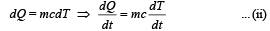From (i) and (ii)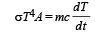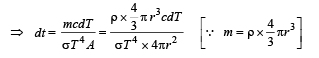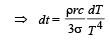Integrating both sides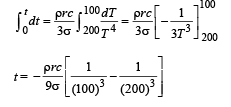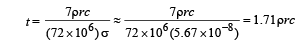Q.7. A point source of heat of power P is placed at the centre of a spherical shell of mean radius R. The material of the shell has thermal conductivity K. If the temperature difference between the outer and inner surface of the shell in not to exceed T, the thickness of the shell should not be less than ............

Ans.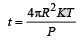Solution.

KEY CONCEPT :

When the spherical shell is thin, t << R. In this case, The rate of flow of heat from the sphere to the surroundings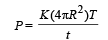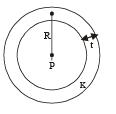where T is the temperature difference and t is the thickness of steel then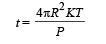Q.8. A substance of mass M kg requires a power input of P watts to remain in the molten state at its melting point. When the power source is turned off, the sample completely solidifies in time t seconds. The latent heat of fusion of the substance is ....................

Ans.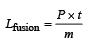Solution. Since P joules per second of heat is supplied to keep the substance in molten state, it means that the substance in the molten state at its melting point releases P Joule of heat in one second.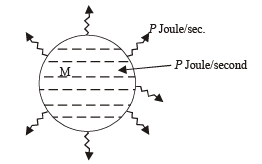The power is turned off then the heat input becomes zero.

But heat output continues. It takes t seconds for the substance to solidify (given). Therefore total heat released in t seconds = P × t = mLfusion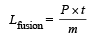Q.9. A container of volume 1m3 is divided into two equal parts by a partition. One part has an ideal gas at 300K and the other part is vacuum. The whole system is thermally isolated from the surroundings. When the partition is removed, the gas expands to occupy the whole volume. Its temperature will now be.......

Ans. 300 K

Solution. In this expansion, no work is done because the gas expands in vacuum. Therefore ΔW = 0

As the process is a adiabatic, Q = 0. From first law of thermodynamics, ΔU = 0 i.e. temperature remains constant.

Q.10. An ideal gas with pressure P, volume V and temperature T is expanded isothermally to a volume 2V and a final pressure Pi. If the same gas is expanded adiabatically to a volume 2V, the final pressure is Pa. The ratio of the specific heats of the gas is 1.67 . The ratio Pa/R is ...

Ans. 0.628

Solution. For isothermal expansion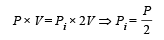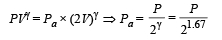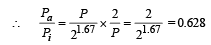Q.11. Two metal cubes A and B of same size are arranged as shown in Figure. The extreme ends of the combination are maintained at the indicated temperatures. The arrangement is thermally insulated. The coefficients of thermal conductivity of A and B are 300 W/m °C and 200 W/m°C, respectively. After steady state is reached the temperature t of the interface will be .....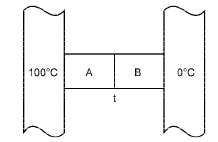Ans. 60°C Solution.

Solution. The heat transferred through A per second

Q1= K1 A (100 – t)

The heat transferred through B per second

Q2= K2 A (t – 0)

At steady state K1 A (100 – t) = K2A (t – 0)

⇒ 300 (100 – t) = 200 (t – 0) ⇒ 300 – 3t = 2t ⇒ t = 60° C

Q.12. A ring shaped tube contains two ideal gases with equal masses and relative molar masses M1= 32 and M2 = 28. The gases are separated by one fixed partition and another movable stopper S which can move freely without friction inside the ring. The angle a as shown in the figure is ........... degrees.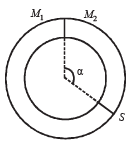Ans. 192º

Solution. The movable stopper will adjust to a position with equal pressure on either sides. Applying ideal gas equation to the two gases, we get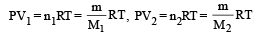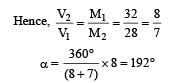Q.13. Earth receives 1400 W/m 2 of solar power. If all the solar energy falling on a lens of area 0.2 m2 is focused on to a block of ice of mass 280 grams, the time taken to melt the ice will be... minutes. (Latent heat of fusion of ice = 3.3 × 105J/ kg.

Ans. 5.5 min.

Solution.  Solar power received by earth = 1400 W/m2

Solar power received by 0.2 m2 area

= (1400 W/m2) (0.2 m2) = 280 W

Mass of ice = 280 g = 0.280 kg

Heat required to meltice .

= (0.280) (3.3 × 105) = 9.24 × 104 J

The document Fill in the Blanks: Heat & Thermodynamics | JEE Advanced Notes | Study Physics 35 Years JEE Main & Advanced Past year Papers - JEE is a part of the JEE Course Physics 35 Years JEE Main & Advanced Past year Papers.
All you need of JEE at this link: JEEUse Code STAYHOME200 and get INR 200 additional OFF Use Coupon Code

Top Courses for JEE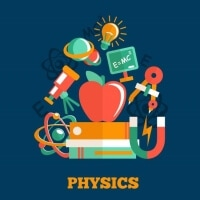Physics 35 Years JEE Main & Advanced Past year Papers

88 docs|49 tests

Top Courses for JEETrack your progress, build streaks, highlight & save important lessons and more!

,

,

,

,

,

,

,

,

,

,

,

,

,

,

,

,

,

,

,

,

,

;